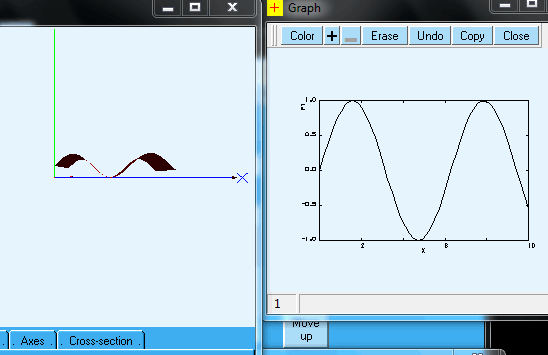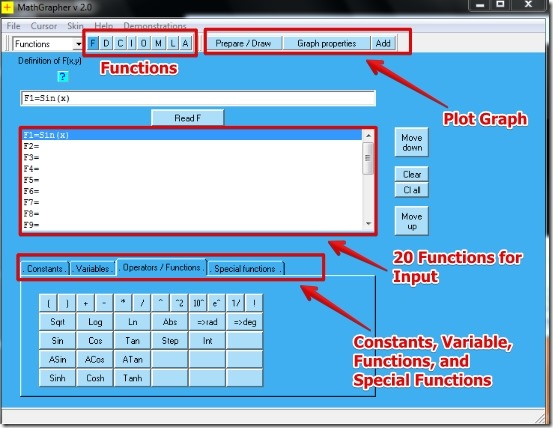Editor Ratings:
User Ratings:
[Total: 0 Average: 0]

MathGrapher is free graphing software using which you can plot mathematical functions in 2D and 3D graphical form. MathGrapher lets you plot the linear and non linear graphs using different mathematical functions.  MathGrapher offers great predefined functions, which you can use for your own mathematical equations.

In MathGrapher you get options for Trigonometric functions, Curve Fitting, Iterations (maps), Ordinary Differential equations, Lindenmayer systems, Cellular automata, Data, and Matrices. Also, the MathGrapher offers special functions like Random, Fresnel, Exponential, and many more. I have tested the MathGrapher in Windows 7 and it worked great for me. I have used MathGrapher to plot graph of Sin(x) both in 2D and 3D graph form for function F1 . See below to get an idea for the 2D and 3D graph that I plotted in MathGrapher.## Working With The MathGrapher To Plot Graphs:

When you start MathGrapher, you see the nice user interface. The User interface is fine and the options are well placed in the menu toolbar.To begin plotting of the graph, you can select either of the function category. You can select from the alphabets F, D, C, I, O, M, L, and A for different functions that MathGrapher offers. After selecting the functions and adding the values, you can select to plot the graph. For the graph you have different options that are:

• Prepare/Draw the Graph – Here you can see the plotted graph. You can plot the graph in 2D and 3D view.
• Graph Properties – Here you can edit the scaling annotation, axis, labels, colors, view Logarithmic scale, and lot more.

MathGrapher lets you save your work for function, data, and image.

## Features of MathGrapher:

• Customizable Graph functions.
• Wide range of graph functions.
• Special functions which include:
• Ran(a) and Random(a,x).
• Modulo function
• Fresnel Integrals:
• Sine Fresnel Integral.
• Cosine Fresnel Integral.
• Exponential integrals and related functions:
• Exponential integral Ei.
• Exponential integral En.
• Logarithmic integral.
• Sine integral.
• Cosine integral.
• Hyperbolic sine integral.
• Hyperbolic cosine integral.
• Gamma function and related functions:
• Gamma function.
• Incomplete gamma function of the first kind.
• Incomplete gamma function of the second kind.
• Riemann Zeta function:
• Zeta function.
• Bessel functions:
• Bessel functions of the first kind.
• Bessel functions of the second kind.
• Elliptic integrals:
• Elliptic integral of the first kind.
• Elliptic integral of the second kind.
• Airy functions:
• Airy functions (first and second kind).
• Probability function:
• Poisson probability function.
• Binomial probability function.
• Distribution functions:
• Binomial distribution function.
• Poisson distribution function.
• Error Function.
• Gaussian distribution function.
• Beta distribution function.
• Chi squared distribution function.
• Student’s t distribution function.
• Option to assign 20 different input functions for graphs.
• View the 2D and 3D orientation of the plotted graph.
• Rotate and zoom while using the mouse for 3D plot.
• Option to save and import the function, data, and graph.
• Great help section.

You might also want to try Graphs Made Easy. It is a free graph making tool that helps you to create attractive graphs.

## Final Verdict:

The MathGrapher is great software to plot graphs. What I really liked is the large predefined and special functions that can be used to plot the graph. Also the 2D and 3D graph plotting is great. Based on the great functions and easy graph plotting I would recommend you to try it out.